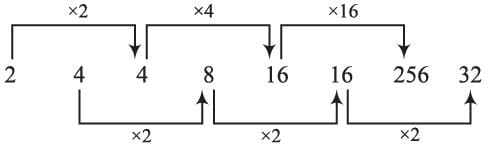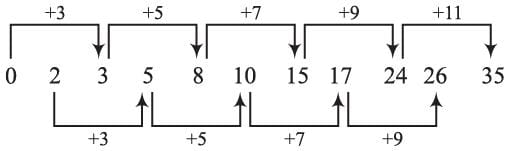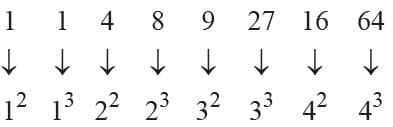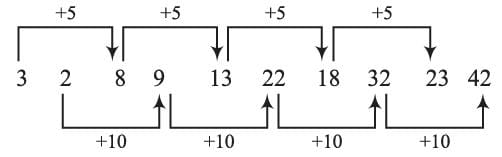## 15 Questions MCQ Test Science Olympiad Class 9 | Olympiad Test: Series Completion

Description
Attempt Olympiad Test: Series Completion | 15 questions in 15 minutes | Mock test for Class 9 preparation | Free important questions MCQ to study Science Olympiad Class 9 for Class 9 Exam | Download free PDF with solutions
QUESTION: 1

### There is a series of numbers which follow some definite order. Find the missing term and complete the series. 3, 4, 5, 5, 12, 13, 7, 24, 25, 9, ?, 41

Solution:

The given numbers are in the set of three numbers which are Pythagorean triplet.
32 + 42 = 52, 52 + 122 = 132,
72 + 242 = 252,  9+ 402 = 412.

QUESTION: 2

### There is a series of numbers which follow some definite order. Find the missing term and complete the series. 10, 17, 26, 37, 50, …. ?

Solution:

The pattern of the given series is
32 + 1 = 10;  42 + 1 = 17;  52 + 1 = 26;
62 + 1 = 37;  72 + 1 = 50;
∴ 82 + 1 = 65.

QUESTION: 3

### There is a series of numbers which follow some definite order. Find the missing term and complete the series. 2, 4, 4, /8, 16, 16, 256, ?

Solution:∴ ? = 32

QUESTION: 4

There is a series of numbers which follow some definite order. Find the missing term and complete the series.

1, 5, 7, 14, 18, 20, 40, 44, 46, ?

Solution:

1 + 4 = 5; 5 + 2 = 7; 7 × 2 = 14
14 + 4  = 18; 18 + 2 = 20; 20 × 2 = 40
40 + 4  = 44; 44 + 2 = 46; 46 × 2 = 92
∴ ? = 92

QUESTION: 5

There is a series of numbers which follow some definite order. Find the missing term and complete the series.

5, 12, 7, 15, 8, 18, 10, ?

Solution:

Second, fourth, sixth terms are multiplies of 3 as, 12, 15, 18 and so on the eight-term will be 21.

QUESTION: 6

There is a series of numbers which follow some definite order. Find the missing term and complete the series.

325, 259, 204, 160, 127, 105, ?

Solution:

325 – 66 = 259; 259 – 55 = 204;
204 – 44 = 160; 160 – 33 = 127;
127 – 22 = 105; 105 – 11 = 94
∴ ? = 94

QUESTION: 7

There is a series of numbers which follow some definite order. Find the missing term and complete the series.

0, 2, 3, 5, 8, 10, 15, 17, 24, 26, ?

Solution:∴ ? = 35

QUESTION: 8

There is a series of numbers which follow some definite order. Find the missing term and complete the series.

120, 99, 80, 63, 48, ?

Solution:

Here
120 – 21 = 99;  99 – 19 = 80; 80 – 17 = 63;
63 – 15 = 48; 48 – 13 = 35
∴ ? = 35

QUESTION: 9

There is a series of numbers which follow some definite order. Find the missing term and complete the series.

1, 1, 4, 8, 9, 27, 16, ?

Solution:∴ ? = 64

QUESTION: 10

There is a series of numbers which follow some definite order. Find the missing term and complete the series.

10, 14, 26, 42, 70, ?

Solution:

10 + 14 + 2 = 26; 14 + 26 + 2 = 42;
26 + 4 2 + 2 = 70;   42 + 70 + 2 = 114.
∴ ? = 114

QUESTION: 11

In each of the following questions, one term in the number series is wrong. Find out the wrong term.

196, 169, 144, 121, 101.

Solution:

196 = 142; 169 = 132; 144 = 122;
121 = 112; 102 = 100;
∴ 101 is wrong term.

QUESTION: 12

In each of the following questions, one term in the number series is wrong. Find out the wrong term.

2, 6, 24, 96, 285, 568, 567

Solution:

2 × 6 – 6 = 6;  6 × 5 – 5 = 25;
25 × 4 – 4 = 96;  96 × 3 – 3 = 285;
285 × 2  – 2 = 568;  568 × 1 – 1 = 567
∴ 24 is odd.

QUESTION: 13

In each of the following questions, one term in the number series is wrong. Find out the wrong term.

93, 309, 434, 498, 521, 533

Solution:

93 + 63 = 309;  309 + 53 = 434;
434 + 43 = 498;  498 + 32 = 525;
525 + 23 = 533
∴ 521 is wrong term.

QUESTION: 14

In each of the following questions, one term in the number series is wrong. Find out the wrong term.

3, 2, 8, 9, 13, 22, 18, 32, 23, 42

Solution:2 + 10 = 12
∴ 9 is the wrong term.

QUESTION: 15

In each of the following questions, one term in the number series is wrong. Find out the wrong term.

8, 13, 21, 32, 47, 63, 83

Solution:

8 + 5 = 13; 13 + 8 = 21;
21 + 11 = 32; 32 + 14 = 46;
46 + 17 = 63;  63 + 20 = 83
∴ 47 is odd.Use Code STAYHOME200 and get INR 200 additional OFF Use Coupon Code